# Bayesian Statistics for Data Science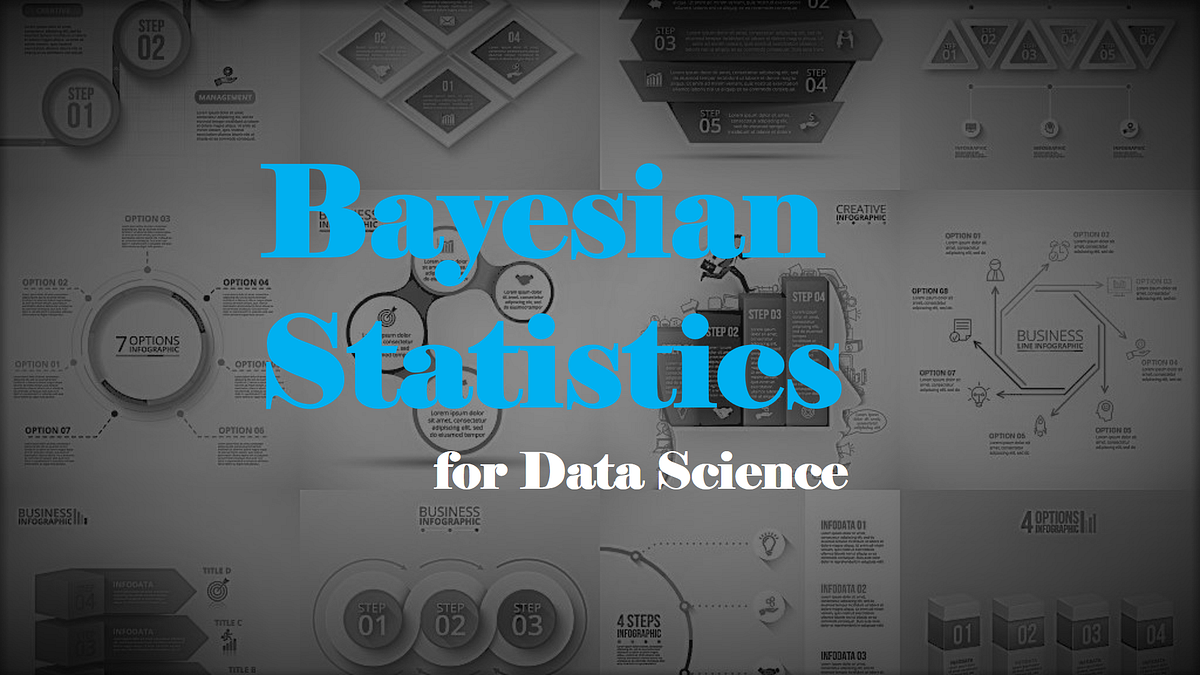This is the 5th post of blog post series ‘Probability & Statistics for Data Science’, this post covers these topics related to Bayesian statistics and their significance in data science.

• Frequentist Vs Bayesian Statistics
• Bayesian Inference
• Test for Significance
• Significance in Data Science

Visit ankitrathi.com now to:

— to read my blog posts on various topics of AI/ML

— to keep a tab on latest & relevant news/articles daily from AI/ML world

— to refer free & useful AI/ML resources

— to buy my books on discounted price

— to know more about me and what I am up to these days

Frequentist Vs Bayesian Statistics

Frequentist Statistics tests whether an event (hypothesis) occurs or not. It calculates the probability of an event in the long run of the experiment. A very common flaw found in frequentist approach i.e. dependence of the result of an experiment on the number of times the experiment is repeated.

Frequentist statistics suffered some great flaws in its design and interpretation which posed a serious concern in all real life problems:

1. p-value & Confidence Interval (C.I) depend heavily on the sample size.
2. Confidence Intervals (C.I) are not probability distributions

Bayesian statistics is a mathematical procedure that applies probabilities to statistical problems. It provides people the tools to update their beliefs in the evidence of new data.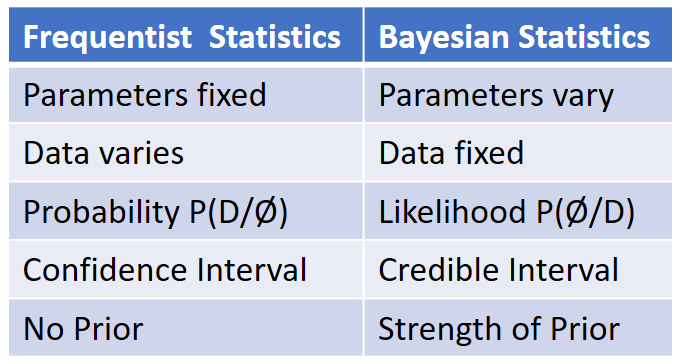[embed]https://www.coursera.org/lecture/bayesian/frequentist-vs-bayesian-inference-q5CTh[/embed]

Bayesian Inference

To understand Bayesian Inference, you need to understand Conditional Probability & Bayes Theorem, if you want to review these concepts, please refer my earlier post in this series.

[embed]https://www.coursera.org/lecture/bayesian/frequentist-vs-bayesian-inference-q5CTh[/embed]

Bayesian inference is a method of statistical inference in which Bayes’ theorem is used to update the probability for a hypothesis as more evidence or information becomes available.

An important part of Bayesian Inference is the establishment of parameters and models. Models are the mathematical formulation of the observed events. Parameters are the factors in the models affecting the observed data. To define our model correctly , we need two mathematical models before hand. One to represent the likelihood function ** and the other for representing the distribution of prior beliefs .** The product of these two gives the posterior belief distribution.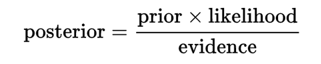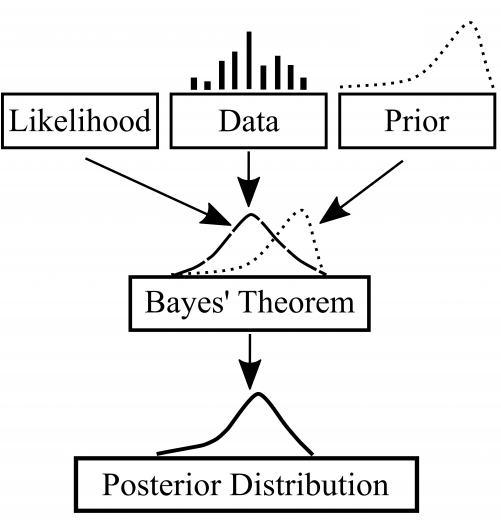Likelihood Function

A likelihood function is a function of the parameters of a statistical model, given specific observed data. Probability describes the plausibility of a random outcome, without reference to any observed data while Likelihood describes the plausibility of a model parameter value, given specific observed data.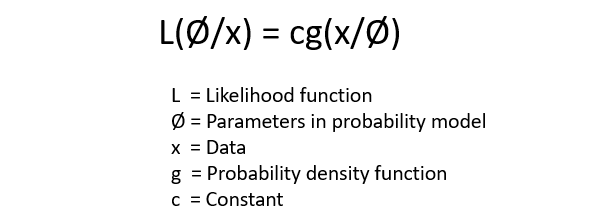[embed]https://www.coursera.org/lecture/bayesian/frequentist-vs-bayesian-inference-q5CTh[/embed]

Prior & Posterior Belief distribution

Prior Belief distribution is used to represent our strengths on beliefs about the parameters based on the previous experience. Posterior Belief distribution is derived from multiplication of likelihood function & Prior Belief distribution.

As we collect more data, our posterior belief move towards prior belief from likelihood: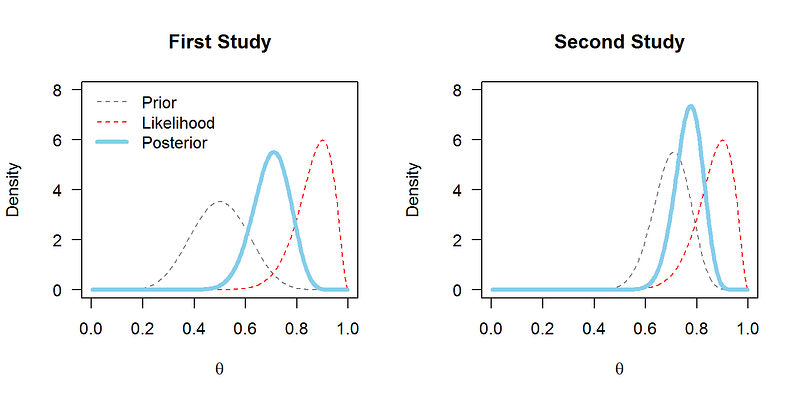[embed]https://www.coursera.org/lecture/bayesian/frequentist-vs-bayesian-inference-q5CTh[/embed]

Test for Significance

Bayes factor

Bayes factor is the equivalent of p-value in the Bayesian framework. The null hypothesis in Bayesian framework assumes ∞ probability distribution only at a particular value of a parameter (say θ=0.5) and a zero probability else where. The alternative hypothesis is that all values of θ are possible, hence a flat curve representing the distribution.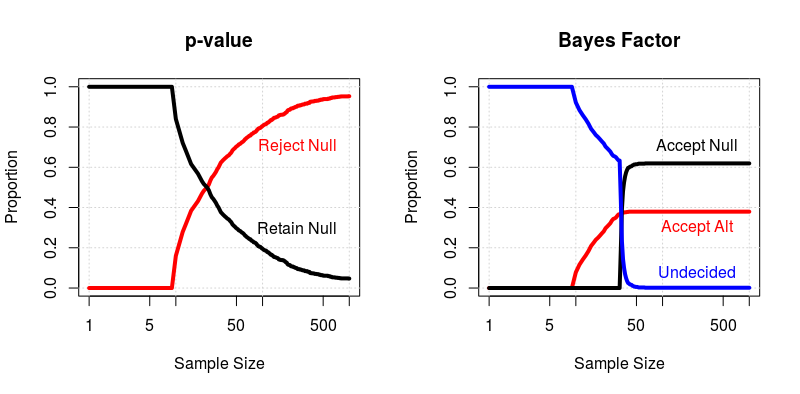Using Bayes Factor instead of p-values is more beneficial in many cases since they are independent of intentions and sample size.

[embed]https://www.coursera.org/lecture/bayesian/frequentist-vs-bayesian-inference-q5CTh[/embed]

High Density Interval (HDI)

High Density Interval (HDI) or Credibility Interval is equivalent to Confidence Interval (CI) in Bayesian framework. HDI is formed from the posterior distribution after observing the new data.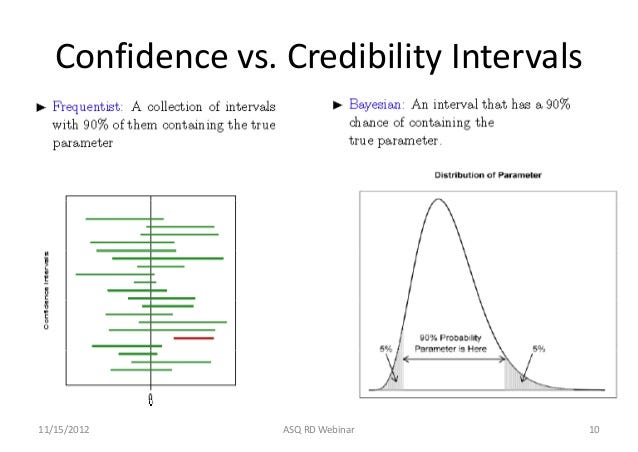Using High Density Interval (HDI) instead of Confidence Interval (CI) is more beneficial since they are independent of intentions and sample size.

[embed]https://www.coursera.org/lecture/bayesian/frequentist-vs-bayesian-inference-q5CTh[/embed]

Moreover, there is a nice article published on AnalyticsVidhya on this which elaborate on these concepts with examples:

[embed]https://www.coursera.org/lecture/bayesian/frequentist-vs-bayesian-inference-q5CTh[/embed]

Significance in Data Science

Bayesian statistics encompasses a specific class of models that could be used for Data Science. Typically, one draws on Bayesian models for one or more of a variety of reasons, such as:

• having relatively few data points
• having strong prior intuitions
• having high levels of uncertainty

And there are scenarios where Bayesian statistics will perform drastically, please read following discussion for details:

[embed]https://www.coursera.org/lecture/bayesian/frequentist-vs-bayesian-inference-q5CTh[/embed]

References:

[embed]https://www.coursera.org/lecture/bayesian/frequentist-vs-bayesian-inference-q5CTh[/embed]
[embed]https://www.coursera.org/lecture/bayesian/frequentist-vs-bayesian-inference-q5CTh[/embed]

Ankit Rathi is an AI architect, published author & well-known speaker. His interest lies primarily in building end-to-end AI applications/products following best practices of Data Engineering and Architecture.